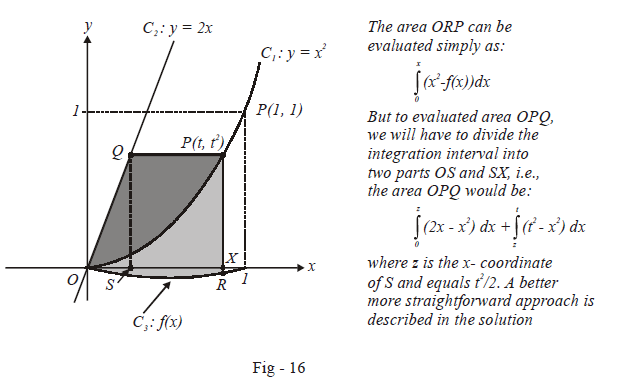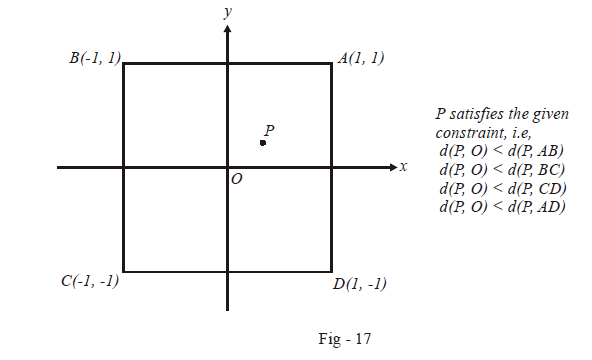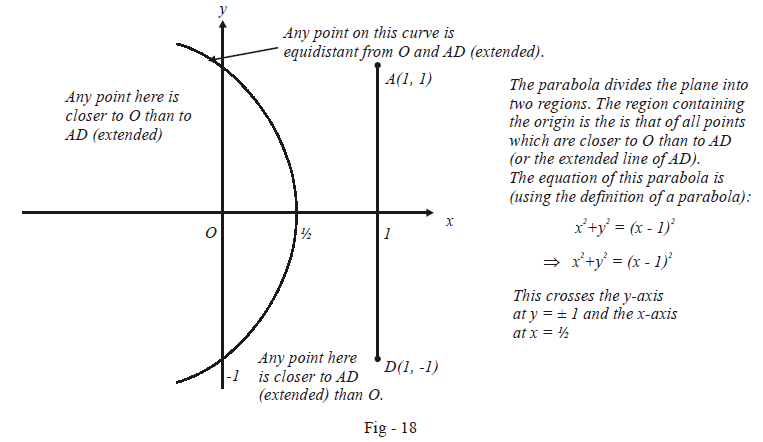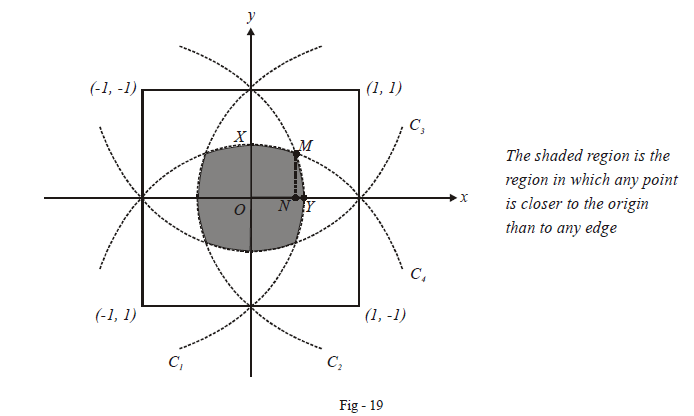# Problems On Areas Set-8

Go back to  'Areas and Volumes'

## Problems on areas

Example – 15

Let $${C_1},{C_2}\;{\rm{and}}\;{C_3}$$ be the graphs of the functions $$y = {x^2},y = 2x$$ , and where $$f\left( 0 \right) = 0$$ .

For a point $$P$$ on $${C_1}$$ , let the lines through $$P$$ parallel to the axes meet $${C_2}\;and\;{C_3}$$ at $$Q$$ and $$R$$ respectively as shown in the figure below. If for every position $$P$$ on $${C_1}$$ , the area of the shaded regions $$OPQ$$ and $$ORP$$ are equal, determine the function $$f\left( x \right)$$ .Solution:

To evaluate area $$OPQ$$, we could follow the approach described above, that is by dividing the integration interval $$OX$$ into $$OS$$ and $$SX$$. By this way,

\begin{align}{\rm{area}}\;OPQ&= \int\limits_0^z {(2x - {x^2})dx + \int\limits_z^1 {({t^2} - {x^2}} } )dx\\& = \left. {\left( {\frac{{{x^2}}}{2} - \frac{{{x^3}}}{3}} \right)} \right|_0^z + \left. {\left( {{t^2}x - \frac{{{x^3}}}{3}} \right)} \right|_z^t\left( {where{\rm{ }}z = \frac{{{t^2}}}{z}} \right)\\& = \frac{{{t^4}}}{8} - \frac{{{t^6}}}{{24}} + {t^2}\left( {t - \frac{{{t^2}}}{2}} \right) - \left( {\frac{{{t^3}}}{3} - \frac{{{t^6}}}{{24}}} \right)\\ &= \frac{{2{t^3}}}{3} - \frac{{{t^4}}}{4}\end{align}

However this area can be more easily evaluated by just considering the inverse of the functions representing $${C_1}\,{\rm{ and}}\,{\rm{ }}{C_2}$$ and integrating with respect to $$y$$. (This means that you view the figure above from left to right inside of the usual top to bottom.) That is, we take $$y$$ as the integration variable. $$y$$ will vary from 0 to $${t^2}$$ (the $$y$$-co-ordinate of $$P$$). The equations of the curves $${C_1}\;{\rm{and }}\;{\rm{ }}{C_2}$$ with respect to the $$y-$$axis will just be the inverse of the original equations i.e. $${C_{{1_y}}} = \sqrt y \;{\rm{ and}} \;{\rm{ }}{C_{{2_y}}} = \frac{y}{2}$$.

Thus,

\begin{align}{\rm{area}}\;OPQ&= \int\limits_0^{{t^2}} {\left( {\sqrt y - \frac{y}{2}} \right)} dy \\& = \left. {\left( {\frac{{2{y^{3/2}}}}{3} - \frac{{{y^2}}}{4}} \right)} \right|_0^{{t^2}}\\ &= \frac{{2{t^3}}}{3} - \frac{{{t^4}}}{4}\end{align}

We see that doing the calculation this way given us the result more quickly because we did not have to divide the integration interval into two sub-intervals as we had to do when we integrated w.r.t x.

The area $$ORP$$ can be easily evaluated (w.r.t the variable $$x$$):

\begin{align}{\rm{area}}\;ORP&= \int\limits_0^t {\left( {{x^2} - f(x)} \right)} dx\\ & = \left. {\frac{{{x^3}}}{3}} \right|_0^t - \int\limits_0^t {f(x)dx} \\& = \frac{{{t^3}}}{3} - \int\limits_0^t {f(x)dx} \end{align}

Since $${\rm{area}}\;OPQ = \;{\rm{area}} \;ORP\quad {\rm{ }}\;\forall {\rm{ }}\quad t \in \left[ {0,{\rm{ }}1} \right],$$ we have

\begin{align}&\qquad\;\;\frac{{2{t^3}}}{3} - \frac{{{t^4}}}{4} = \frac{{{t^3}}}{4} - \int\limits_o^t {f(x)dx} \\&\Rightarrow \quad \int\limits_0^t {f(x)dx = \frac{{{t^4}}}{4}} - \frac{{{t^3}}}{3}\end{align}

Differentiating both sides w.r.t t, we obtain our required function :

$f(t) = {t^3} - {t^2}$

Thus, the curve $${C_3}$$ is given by $$f\left( x \right) = {x^3} - {x^2}{\rm{ }}in{\rm{ }}\left[ {0,{\rm{ }}1} \right]$$ .

Example – 16

Consider a square with vertices at $$\left( {1,{\rm{ }}1} \right),{\rm{ }}( - 1,{\rm{ }}1),{\rm{ }}( - 1, - 1){\rm{and }}\;{\rm{ }}(1, - 1)$$ . Let $$S$$ be the region consisting of all points inside the square which are nearer to the origin than to any edge. Sketch the region $$S$$ and find its area.

Solution: The important part in this question is to correctly plot the required region. Once that is done, the area is more or less straight forward to evaluate. Consider the square $$ABCD$$ described in the question and any point $$P$$ inside it satisfying the given constraint.Consider the point $$P$$ and any one edge of the square, say $$AD.$$ Suppose $$P$$ was equidistant from $$O$$ and $$AD$$ (or its extended line). Where could it possible lie? What is the locus of a point which moves so that its distance from a fixed point is equal to its distance from a fixed line? A parabola.

Since $$d (P,O) < d (P, AD)$$, the point $$P$$ will lie not on the parabola but in one of the regions that the parabola divides the plane into:We will now have to draw such a parabola for each edge of the square since we want that each edge should satisfy the given constraint, that is any point in the required region should lie closer to the origin than to any edge:Verify that the equations of the other three bounding parabolas (apart from $${C_1}$$ ) are :

$\begin{array}{l}{C_2}:{y^2} = 2x + 1\\{C_3}:{x^2} = 2y + 1\\{C_4}:{x^2} = - 2y + 1\end{array}$

To calculate the required area, let us calculate the shaded region in the first quadrant. We can divide this area into two parts: area (region $$OXMN$$) and area (region $$NMY$$).

We first need the intersection point $$M$$ and its $$x-$$co-ordinate $$N$$. This can be found out by equating the equations of the curves $${C_1}{\rm{ and}}\;{\rm{ }}{C_4}.$$ However, we may directly observe that the point $$M$$ lies symmetrically w.r.t the $$x-$$axis and the $$y-$$axis, and therefore, it will have equal $$x$$ and $$y$$  co-ordinates. We may now use this fact in either of the two equations $${C_1}{\rm{ }}\;and\;{\rm{ }}{C_4}$$ :

\begin{align}&{C_4}:{x^2} + 2y - 1 = 0\\&\Rightarrow \quad {x^2} + 2x - 1 = 0 \qquad {\rm{(\because\ x = y for M)}}\\&\Rightarrow \quad x{\rm{ = }}\sqrt 2 - 1 \qquad \;\qquad{\rm{(we\; want}} \;x > 0)\end{align}

Thus, the point $$M$$ is $$\left( {\sqrt 2 - 1,{\rm{ }}\sqrt 2 - 1} \right).$$

We now proceed to evaluate the area.

\begin{align}{A_{1stquad}} &= \int\limits_0^{\sqrt 2 - 1} {\left( {\frac{{1 - {x^2}}}{2}} \right)} dx + \int\limits_{\sqrt 2 - 1}^1 {\sqrt {1 - 2x} } dx\\&{\rm{ }}\qquad \quad{ \uparrow _{Equation\;of\;{C_4}}}\qquad \qquad{\rm{ }}{ \uparrow _{Equation\;of\;{C_1}}}\\\\ & {\left. {= \left( {\frac{x}{2} - \frac{{{x^3}}}{6}} \right)} \right|_0}^{\sqrt {2 - 1} }{\left. { - \frac{1}{3}\left( {{{\left( {1 - 2x} \right)}^{3/2}}} \right)} \right|^1}_{\sqrt {2 - 1} }\\\\& = \frac{1}{2}\left( {\sqrt 2 - 1 - \frac{{{{(\sqrt 2 - 1)}^3}}}{3}} \right) - \frac{1}{3}\left( {0 - {{\left( {3 - 2\sqrt 2 } \right)}^{3/2}}} \right) \\& = \frac{{(\sqrt 2 - 1)}}{2} + \frac{{{{(\sqrt 2 - 1)}^3}}}{6} + \frac{{{{(\sqrt 2 - 1)}^3}}}{3} \\\\& = \frac{{(\sqrt 2 - 1)(3 - \sqrt 2 )}}{3} \end{align}

Thus, the total required area is

$A = \frac{{4\left( {\sqrt 2 - 1} \right)\left( {3 - \sqrt 2 } \right)}}{3}$